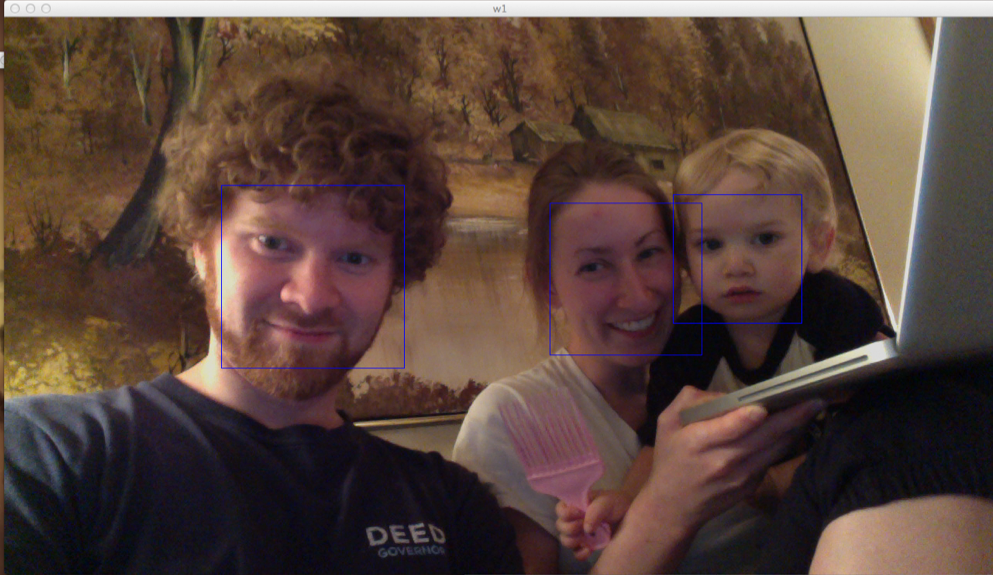# Facial Detection with OpenCV and Python

I was able to get some basic facial detection working in OpenCV with Python. Here's what it looks like:And here is the 34-line python script to draw boxes around all detected faces in live video:

import cv

CAMERA_INDEX = 0

def detect_faces(image):
faces = []
detected = cv.HaarDetectObjects(image, cascade, storage, 1.2, 2, cv.CV_HAAR_DO_CANNY_PRUNING, (100,100))
if detected:
for (x,y,w,h),n in detected:
faces.append((x,y,w,h))
return faces

if __name__ == "__main__":
cv.NamedWindow("Video", cv.CV_WINDOW_AUTOSIZE)

capture = cv.CaptureFromCAM(CAMERA_INDEX)
storage = cv.CreateMemStorage()
faces = []

i = 0
while True:
image = cv.QueryFrame(capture)

# Only run the Detection algorithm every 5 frames to improve performance
if i%5==0:
faces = detect_faces(image)

for (x,y,w,h) in faces:
cv.Rectangle(image, (x,y), (x+w,y+h), 255)

cv.ShowImage("w1", image)
i += 1